• 使用逻辑回归预测Titanic幸存者是否幸存，基于Anaconda+juypters实现，参考来自https://github.com/HanXiaoyang/Kaggle_Titanic/blob/master/Titanic.ipynb
• 基于逻辑回归预测贷款违约 摘要 金融机构审批贷款时会收集客户个人信息，包括年龄、收入、学历、职业、家庭情况、借贷历史等信息，在对各项信息综合考虑的基础上决定是否审批贷款。过去该项工作主要依靠人工审核...
                            基于逻辑回归预测贷款违约
摘要
金融机构审批贷款时会收集客户个人信息，包括年龄、收入、学历、职业、家庭情况、借贷历史等信息，在对各项信息综合考虑的基础上决定是否审批贷款。过去该项工作主要依靠人工审核，随着人工智能技术的发展，数据分析和机器学习成为金融风控领域的重要工具。本文利用数据竞赛平台Kaggle提供的贷款历史数据，对数据进行预处理后，使用python构建逻辑回归模型，利用模型预测贷款人的违约情况。
关键词：数据分析，逻辑回归，预测
本文尝试使用python构建模型，利用模型预测贷款人的违约情况。通过贷款数据（如用户个人信息、贷款状态等）来训练模型，通过模型分析贷款人的偿还能力，预测贷款申请人是否会发生违约。因为已知贷款状态是否违约以及各特征变量的值，判定贷款申请人会不会违约是一个二分类问题，是一个监督学习的场景，可以通过分类算法来处理。
本文采用kaggle提供的数据，包含大于284807条用户贷款数据。具体如图1。Class是因变量，0表示没有违约，1表示发生了违约，其他的是自变量。

图1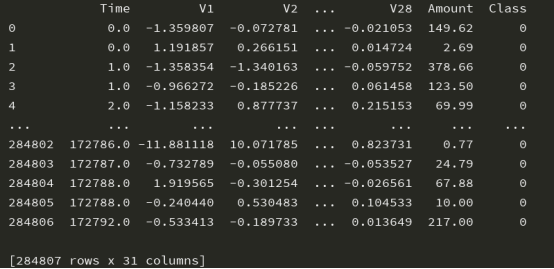数据处理
1.1数据读取与分析
图2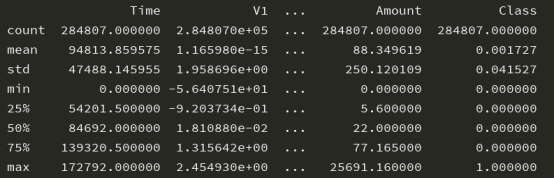Class的均值是0.001727，说明违约率是0.1727%，说明数据不均衡，违约的数据量远远小于正常的数据量，需要进行样本均衡化;Amount变量范围较大，远远大于V1-V28，需要进行标准化处理。Time变量无实际意义，丢弃即可。
1.2.特征标准化
特征标准化就是将数据处理后在一个小范围内波动，公式如下：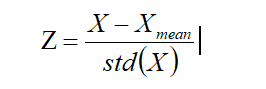Z为标准化后的数据，X为原始数据，Xmean为原始数据的均值，std(X)为原始数据的标准差。Python中提供了StandardScaler模块可以进行特征标准化。
1.3样本均衡化
图3是Class=0和Class=1的数据对比，可以发现正常数据远远超过异常数据，样本存在不均衡现象。

图3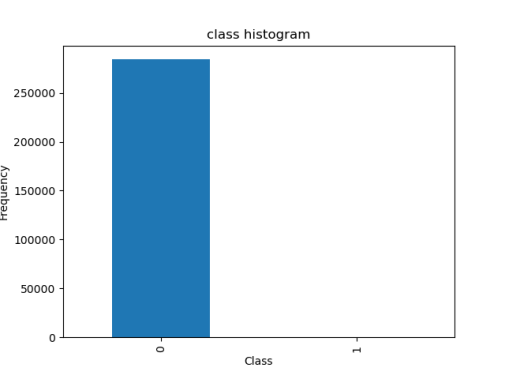样本不均衡的解决方法分为下采样和过采样，下采样会误杀样本数量，使用过采样解决样本不均衡，使用SMOTE算法生成数据，python中提供了imblearn包可以实现该算法。
oversampler=SMOTE(random_state=0)
X_train,y_train=oversampler.fit_sample(X_train,y_train)

2.建立逻辑回归模型
按照80%是比例，把数据分为训练集和测试集。
X_train,X_test,y_train,y_test=train_test_split(X,y,test_size=0.2,random_state=0)
logic_clf=LogisticRegression()  #生成一个逻辑回归模型
logic_clf.fit(X_train,y_train.values.ravel()) #用训练集进行拟合，获得模型
score=logic_clf.score(X_test,y_test) 使用模型对X_test数据进行测试，并与y_test比较，获得准确率
使用confusion_matrix方法获得混淆矩阵 y_pred=logic_clf.predict(X_test)
cnf_matrix=confusion_matrix(y_test,y_pred)
准确率和混淆矩阵如下：
0.9450257636776112
[[55300  1424]
[ 4828 52174]]
3.结论
使用逻辑回归模型，得到的预测准确率94.5%，具有很高的使用价值，在后续的工作中，将尝试采用其他算法例如贝叶斯模型、神经网络的方法构建模型，进一步提高模型准确度。

代码：
import pandas as pd
print(data)

#查看数据概述
print(data.describe())

# #查看样本数据是否均衡
# import matplotlib.pyplot as plt
# count_class=pd.value_counts(data['Class'],sort=True).sort_index()
# count_class.plot(kind='bar')
# plt.title('class histogram')
# plt.xlabel('Class')
# plt.ylabel('Frequency')
# plt.show()

#特征标准化
from sklearn.preprocessing import StandardScaler
data['normAmount']=StandardScaler().fit_transform(data['Amount'].values.reshape(-1,1))
data=data.drop(['Time','Amount'],axis=1)
print(data)

#切分数据
#数据
X=data.loc[:,data.columns!='Class']
#标签
y=data.loc[:,data.columns=='Class']

#过采样
from imblearn.over_sampling import SMOTE
oversampler=SMOTE(random_state=0)
X,y=oversampler.fit_sample(X,y)
print("过采样")
print(X)

from sklearn.model_selection import train_test_split
X_train,X_test,y_train,y_test=train_test_split(X,y,test_size=0.2,random_state=0)
print(X_train)

# #过采样方案
# from imblearn.over_sampling import SMOTE
# oversampler=SMOTE(random_state=0)
# X_train,y_train=oversampler.fit_sample(X_train,y_train)
# print(X_train)

from sklearn.linear_model import LogisticRegression
logic_clf=LogisticRegression()  #生成一个逻辑回归模型
logic_clf.fit(X_train,y_train.values.ravel()) #训练
y_pred=logic_clf.predict(X_test)
#计算准确率
score=logic_clf.score(X_test,y_test)
print(score)

#计算混淆矩阵
from sklearn.metrics import confusion_matrix
cnf_matrix=confusion_matrix(y_test,y_pred)
print(cnf_matrix)

展开全文数据分析 数据分析论文 pythonpython
• SAS EM（四）逻辑回归预测模型 作为某商品类目公司的市场分析师，你可能需要为二值目标变量（purchase）建立逻辑回归模型，判断某个顾客是否会产生购买。输入数据集存放在 SAS 安装文件的 SAMPSIO.DMEXA1中，该数据...
SAS EM（四）逻辑回归预测模型
作为某商品类目公司的市场分析师，你可能需要为二值目标变量（purchase）建立逻辑回归模型，判断某个顾客是否会产生购买。输入数据集存放在 SAS 安装文件的 SAMPSIO.DMEXA1中，该数据集包含 1966 个顾客观测值信息。它包含 31 个区间变量输入和 18 个分类变量输用于构建模型。该逻辑回归模型产生的打分公式应用在新的数据集中，给那些可能产生购买的顾客发送邮件。
创建流程图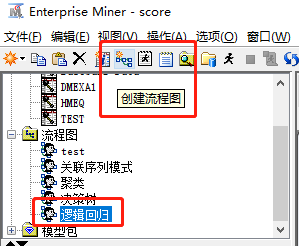创建数据源输入数据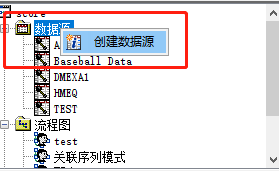找到系统内置的表 SAMPSIO.DMEXA1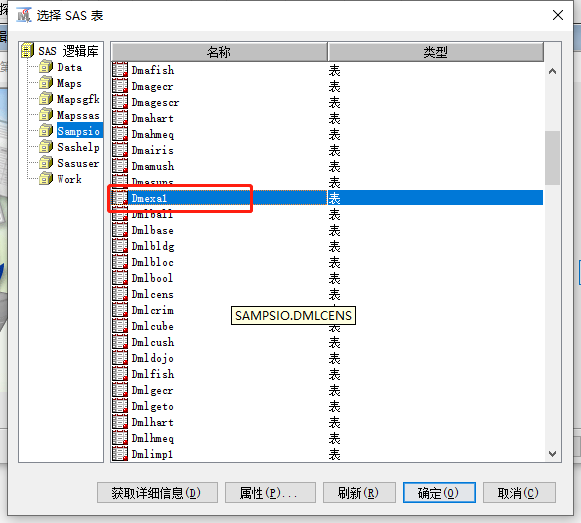数据分区
对输入数据集运用层次抽样方法创建训练和验证数据集。训练数据集被用来拟合回归模型，验证数据集用来评估回归模型。层次抽样技术保留购买者和非购买者在训练集和验证集中的初时比率。 首先，在流程图空间加入数据划分节点，打开数据划分节点，在划分选项卡，设置输入数据集的 70%作为训练集，30%作为验证集，测试为 0。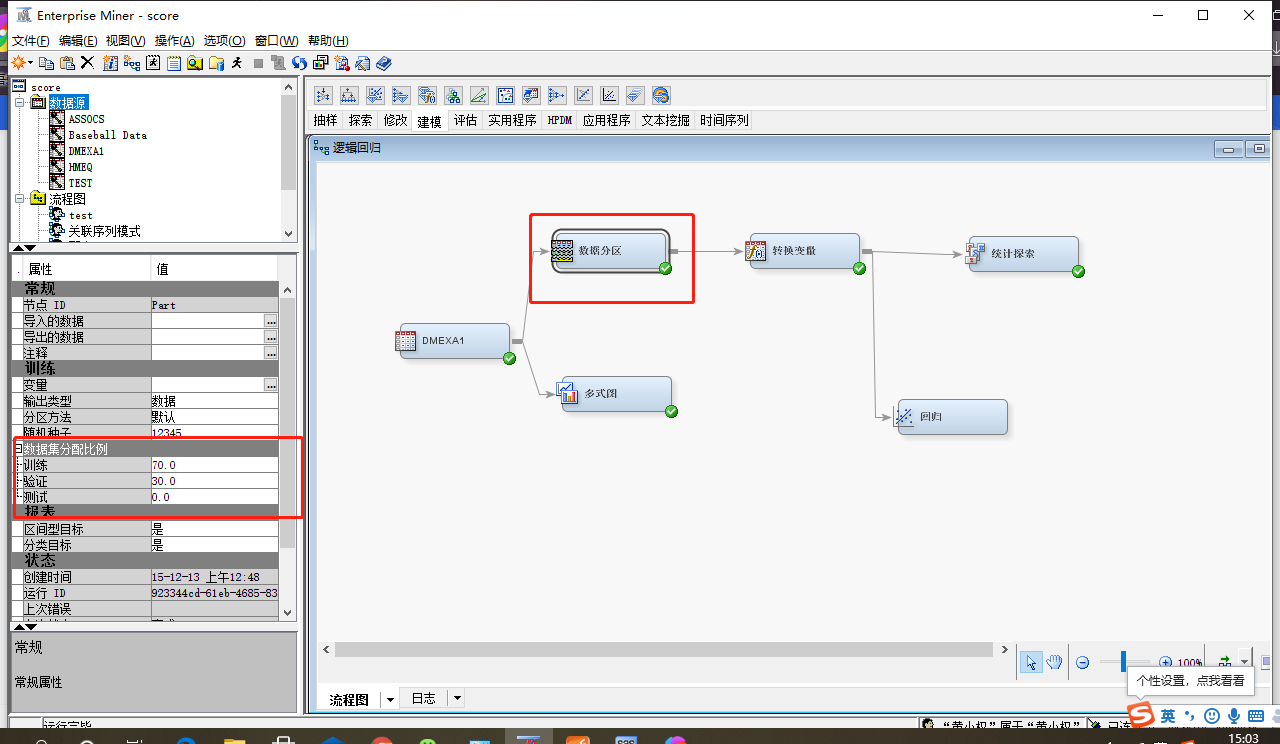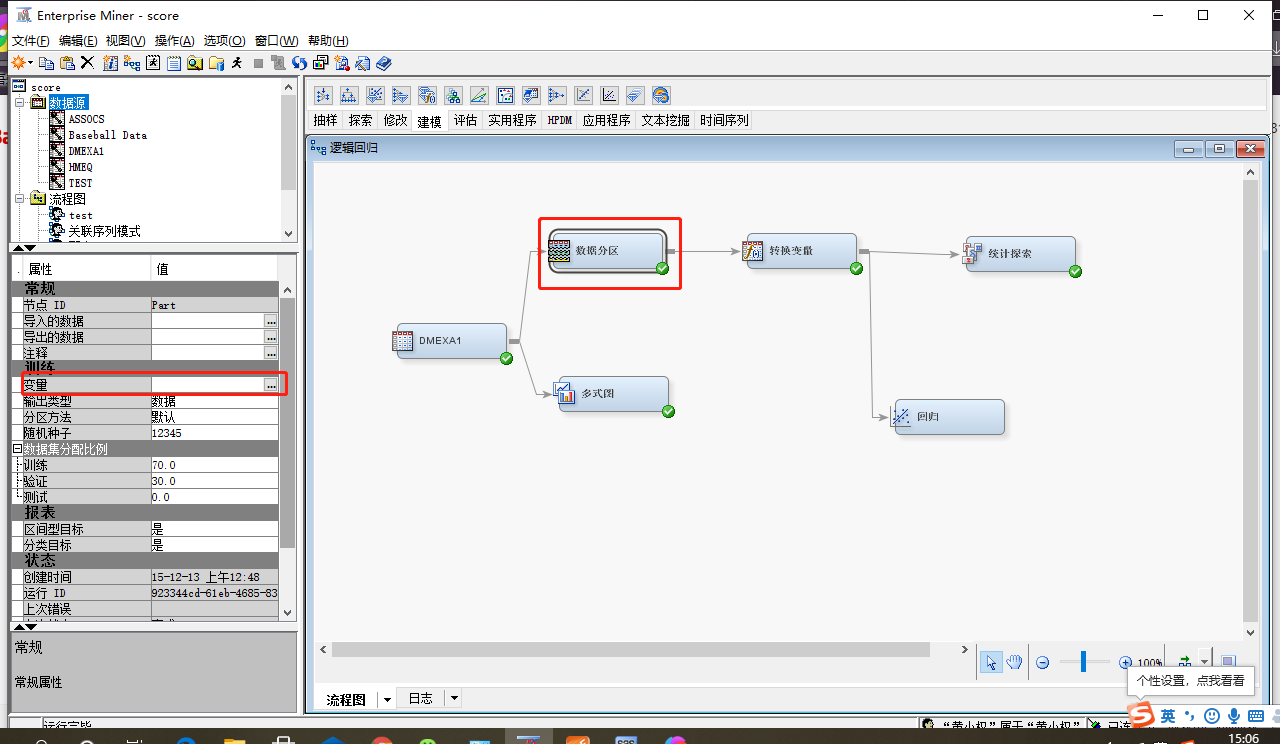针对类型输入变量 STATECOD，选择层次抽样方法单选按钮。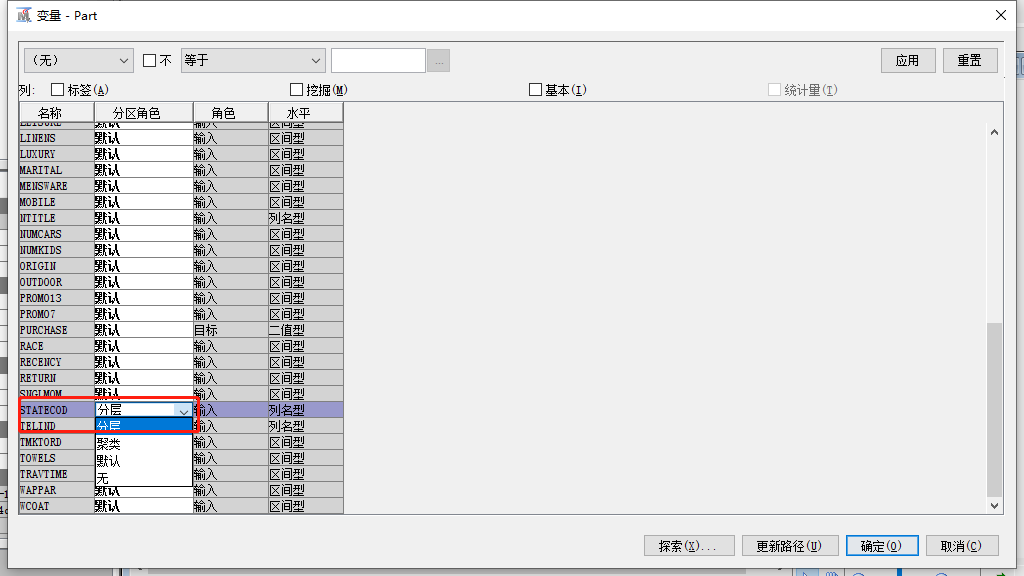转换变量（新增变量，变量log转换，交互变量，变量分箱）
打开转换节点，创建二值变量 wealth，当 income 大于 40000 同时 homeval 大于 100000，则其值为 1，否则为 0。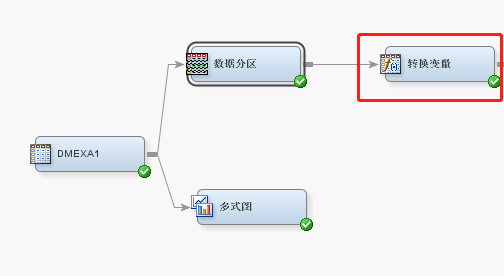从图中可以发现，frequent 的倾斜度很大，极值会影响参数的预测。 右击 frequent，选择 transform，选择方法 log，进行转换。再点击查看变量的分布。转换前及转换后数据分布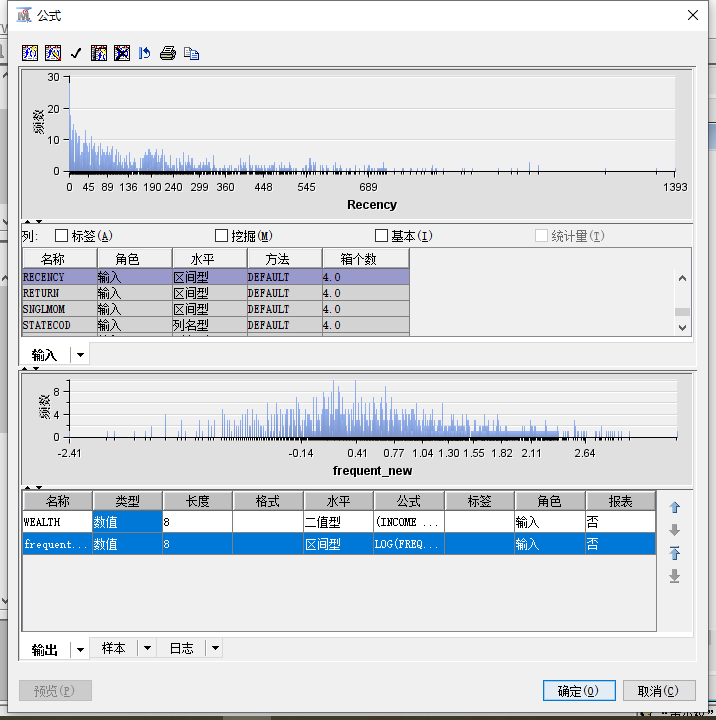我们根据业务经验衍生变量，（转换变量---交互效应）产生新的变量 recency * recency；然后，选择 recency和 ntitle，点击 保存，产生新变量 recency * ntitle。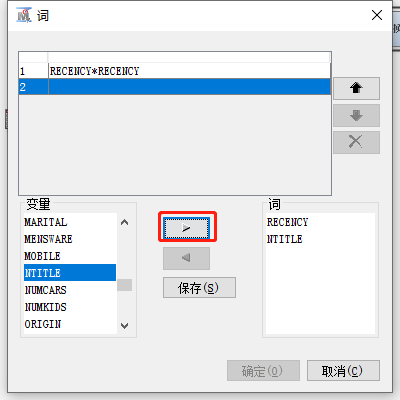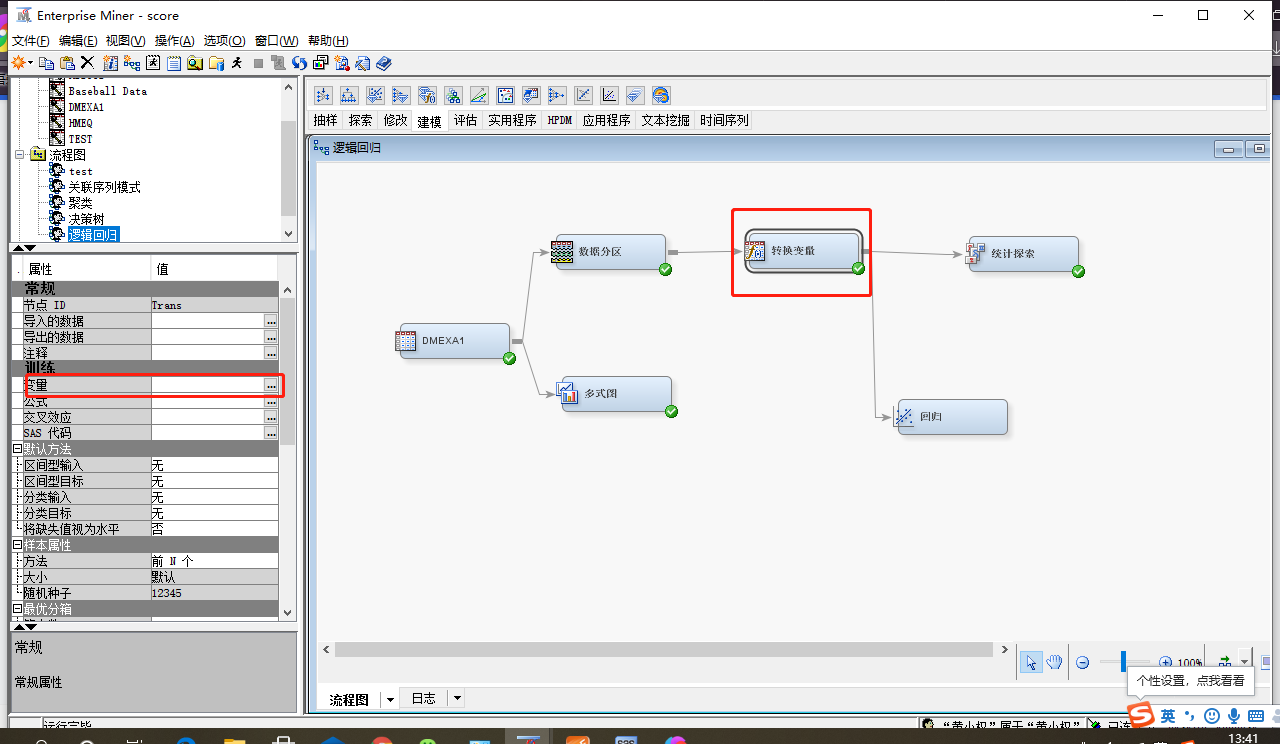创建新的分类变量，对age进行分箱处理，划分为4个年龄组。右击age变量，选择转换变量节点，选择 桶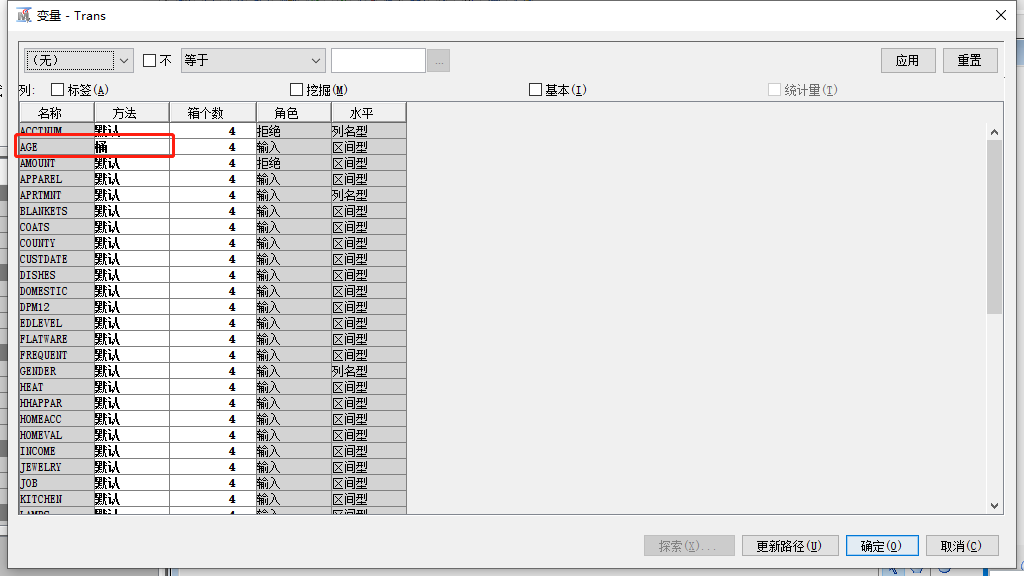查看上面转换变量转换的几个变量详细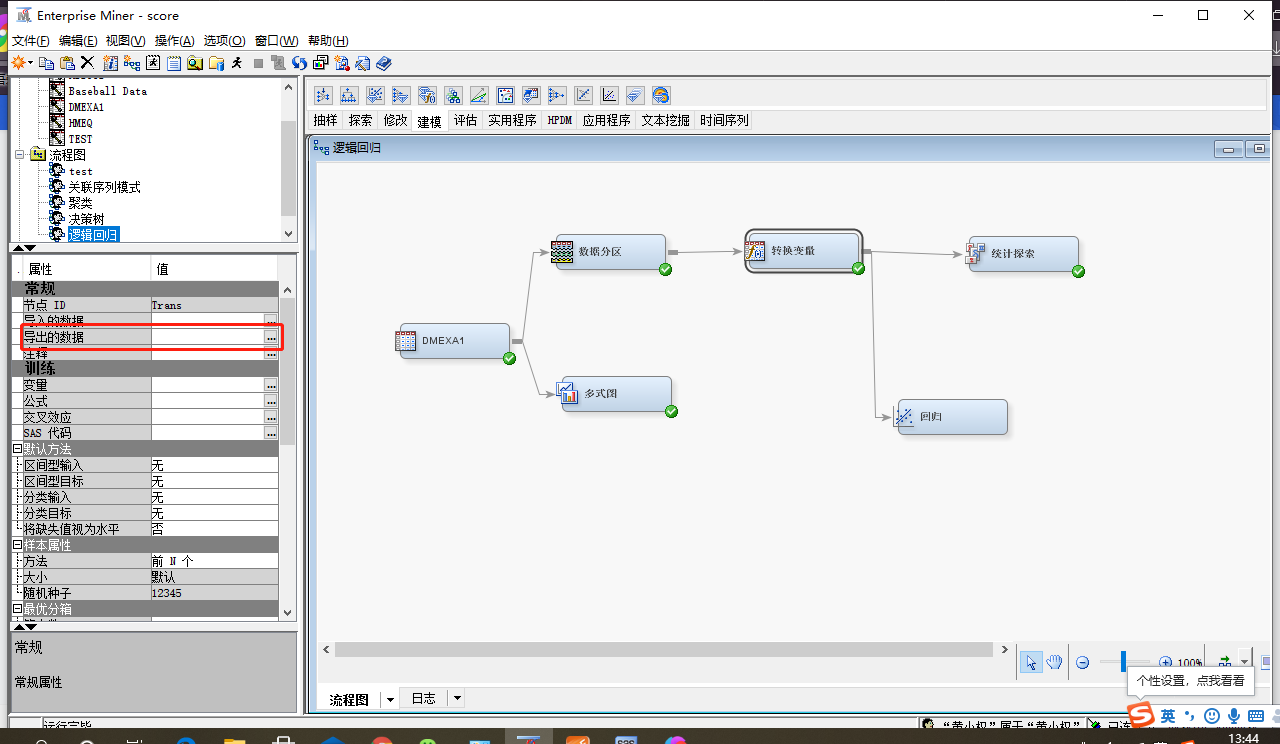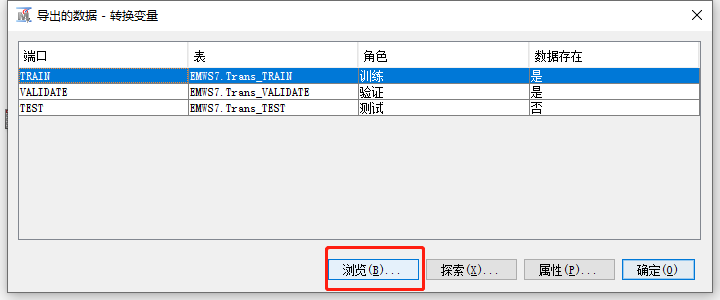这些就是我们刚才在变量转换产生的新变量了，后续用于逻辑回归模型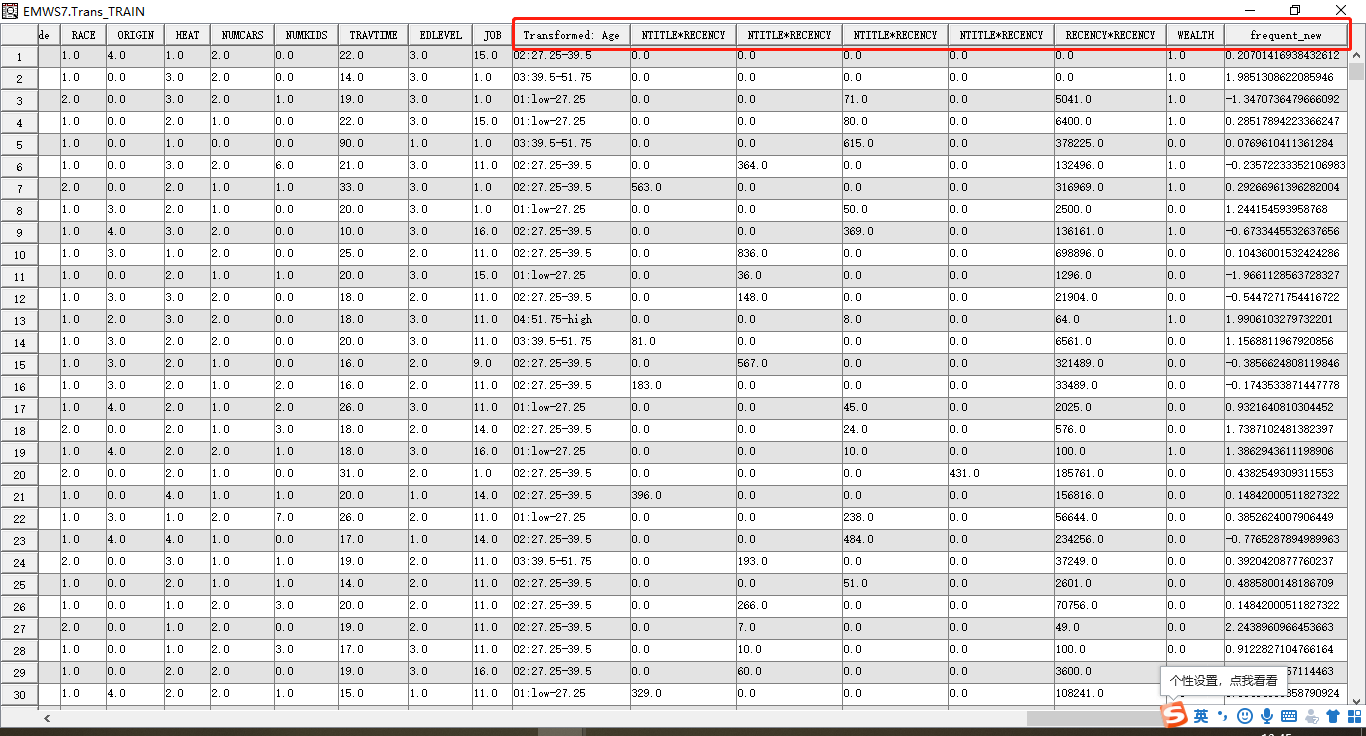统计探索变量
在输入数据源节点，可以在区间变量和分类变量选项卡中，查看观测值的缺失值，在本案例中发现，没有缺失值。在实际中，若存在缺失值，可以加入 replacement 节点，进行处理。在回归模型中不能直接处理缺失值观测值。（下面为查看有无缺少值）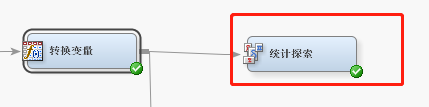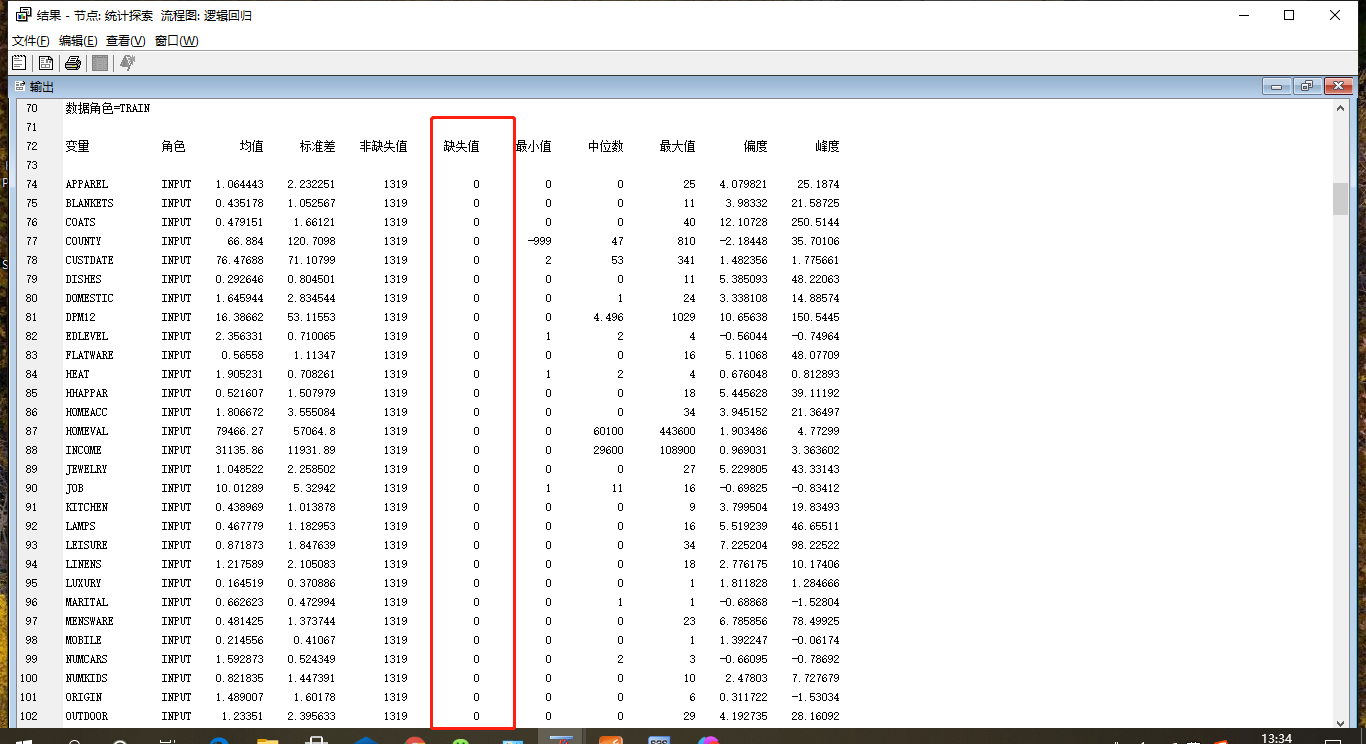回归建模
建立逻辑回归预测模型
选择模型适用逐步回归
设置引入”显著性水平"及“保留”显著性水平为0.1
最大步数为100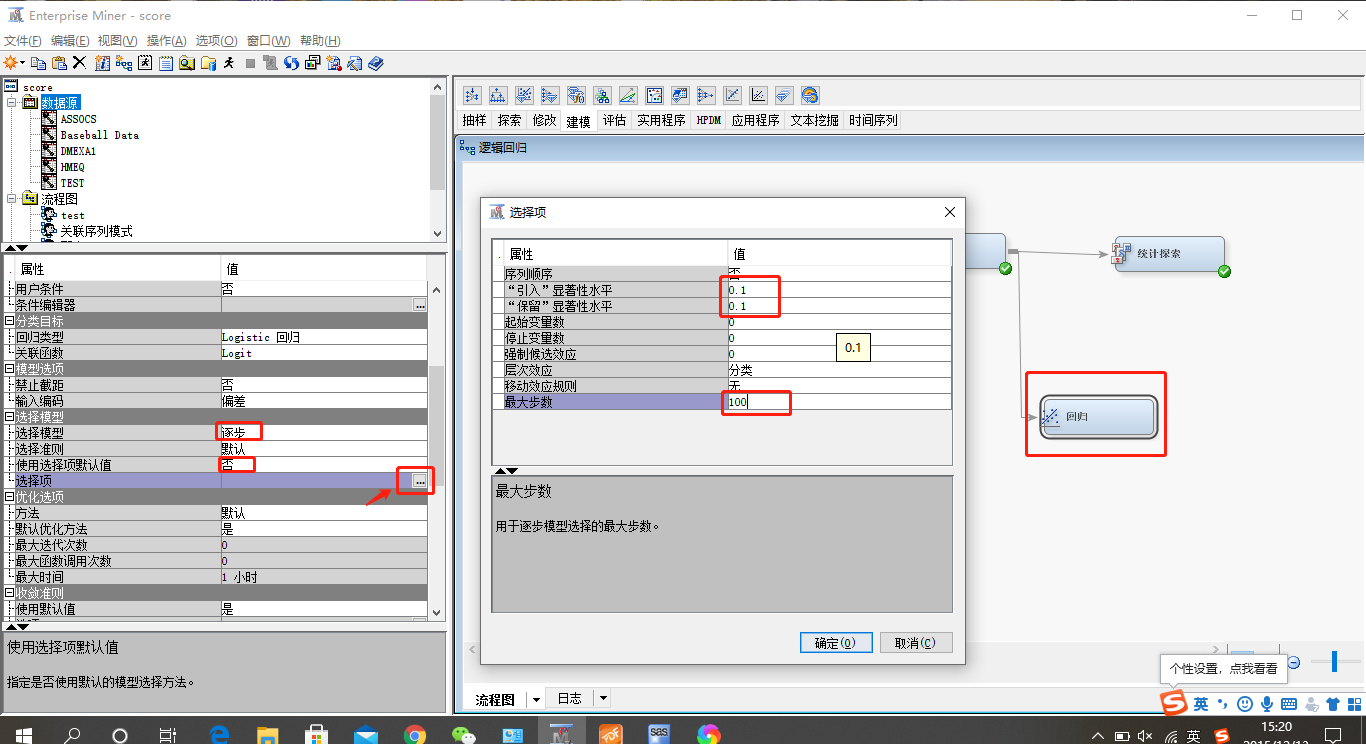结果可关注变量相关性，系数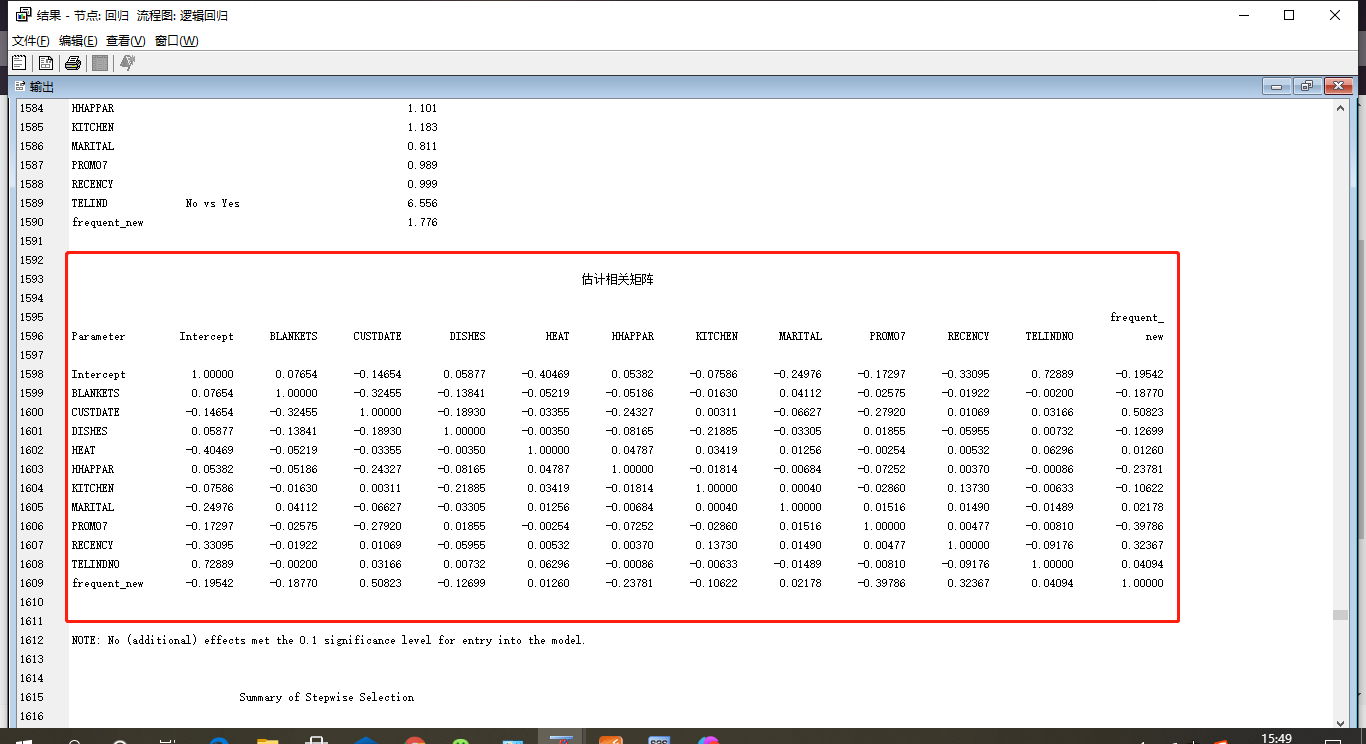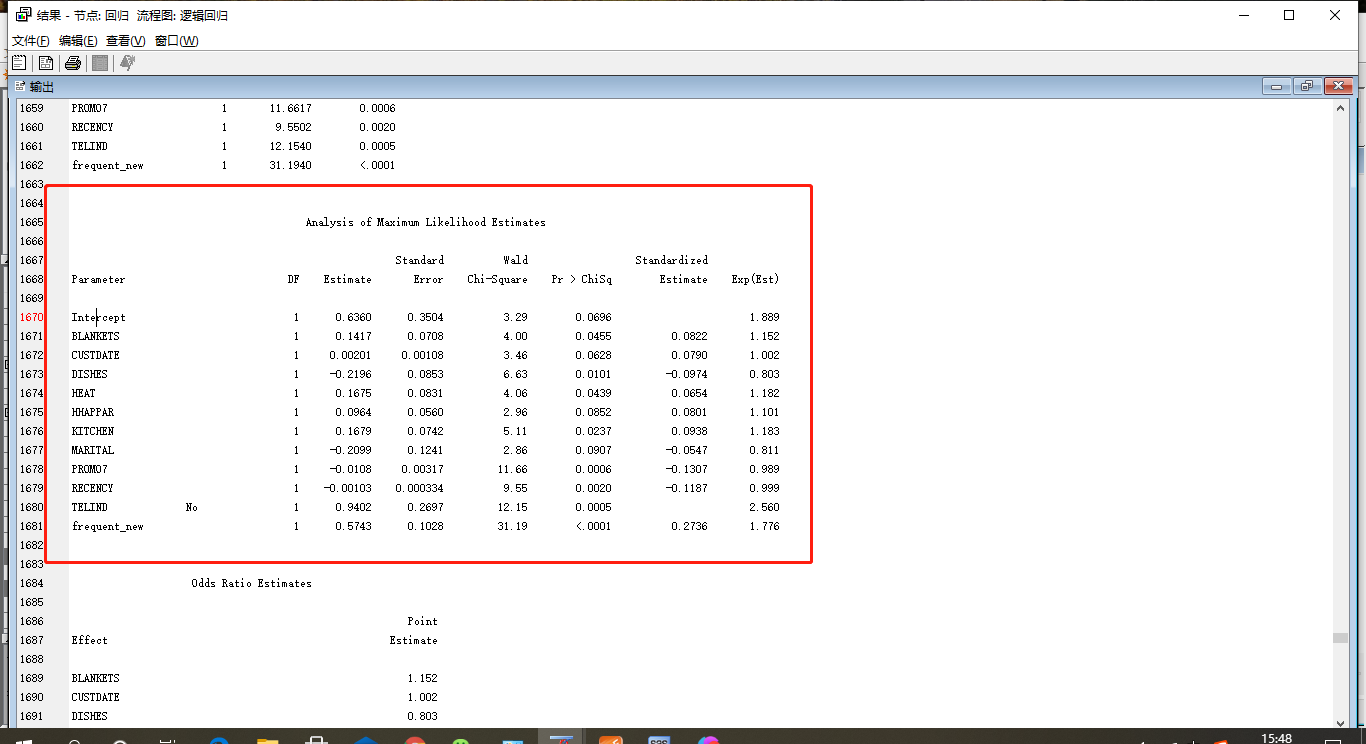也可查看评分分布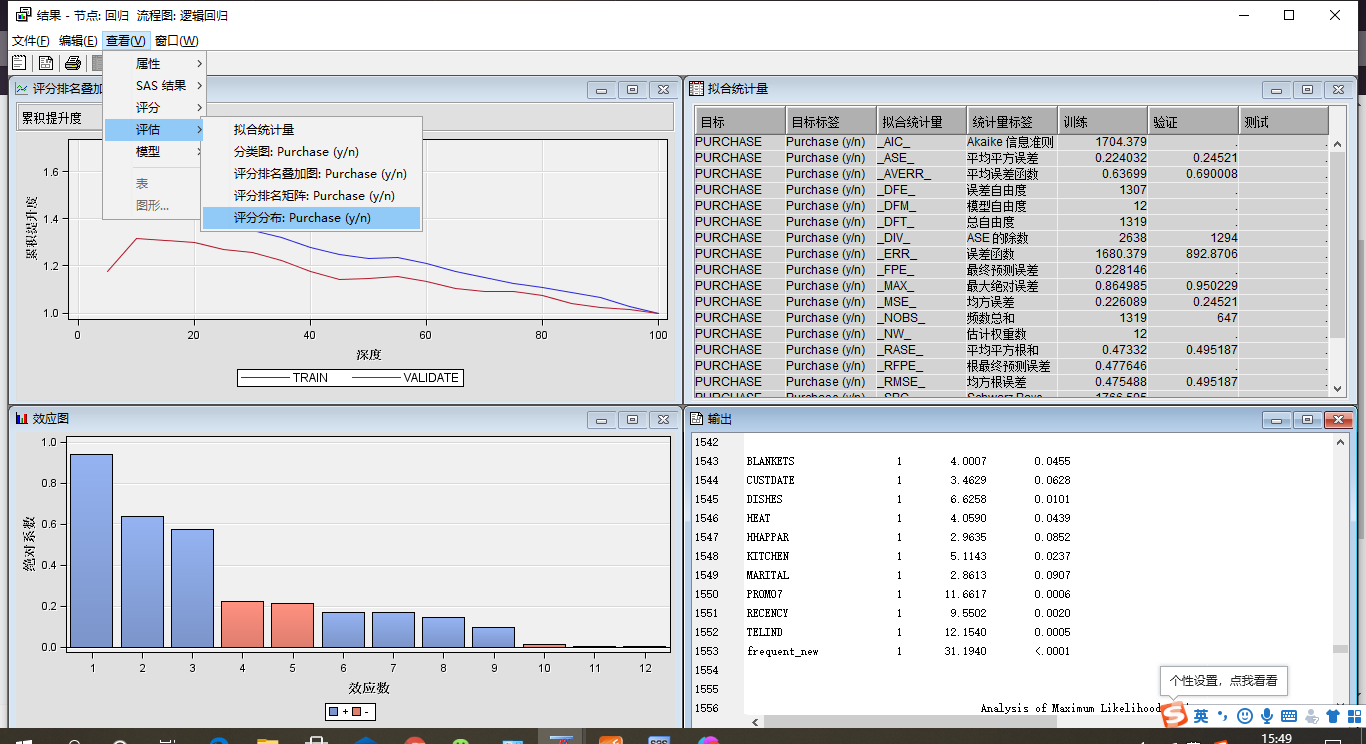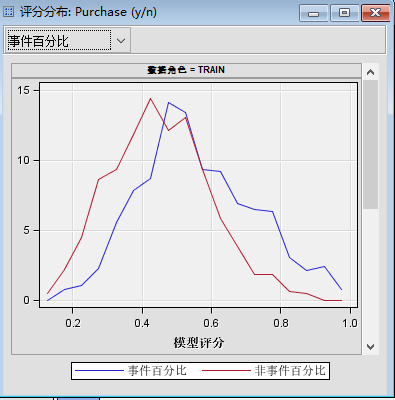评价模型相关指标（KS、ROC）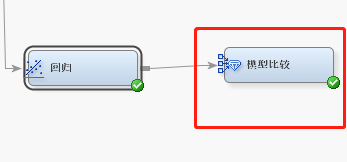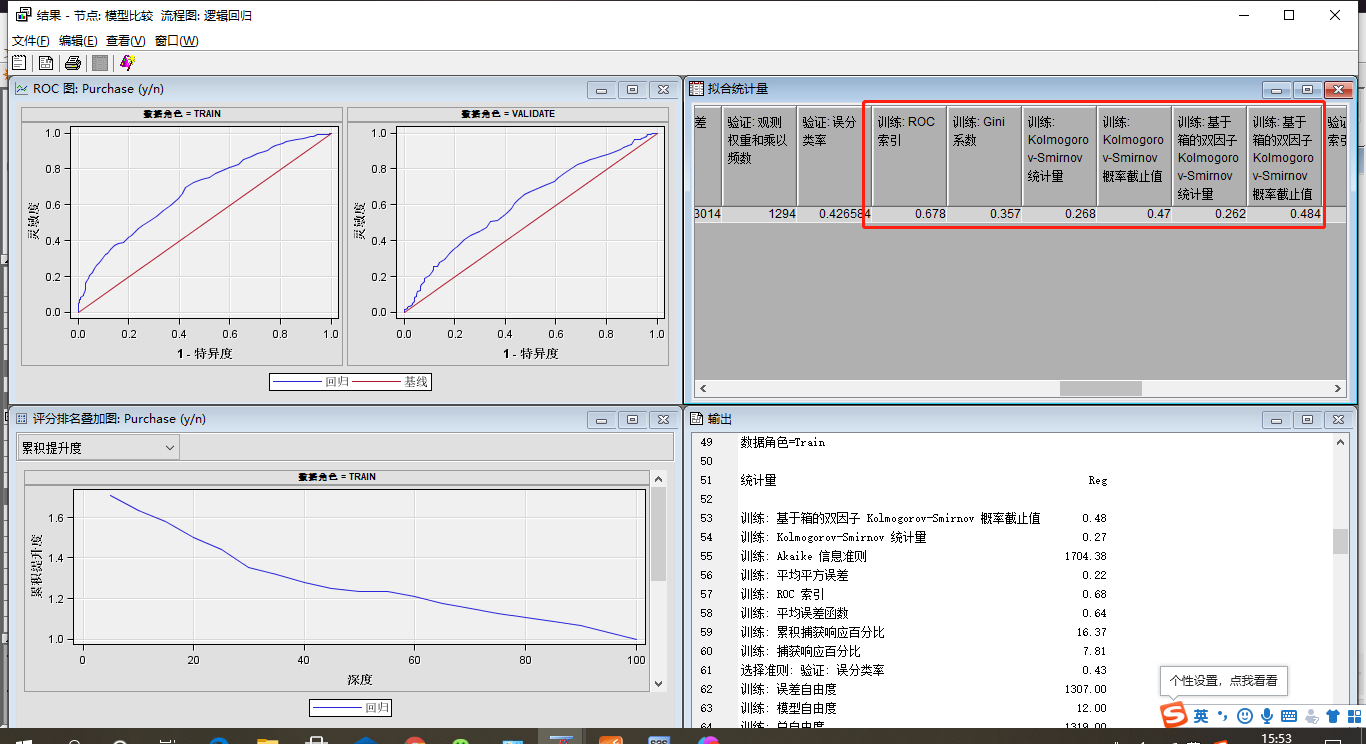预测数据（用原来数据模拟现有数据预测）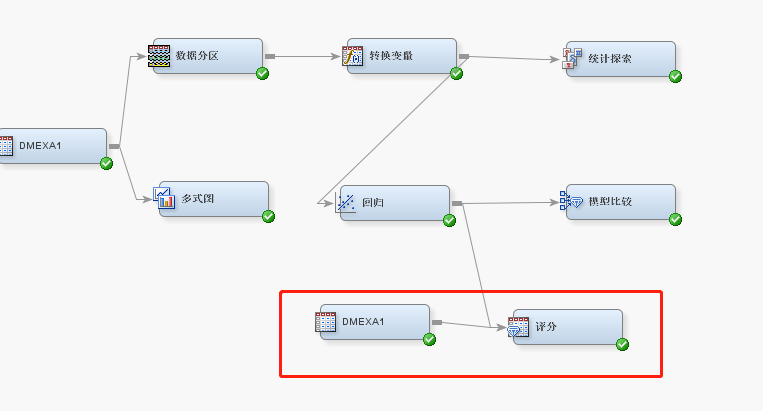新建输入源节点，角色选择为评分，数据源为SAMPSIO.DMEXA1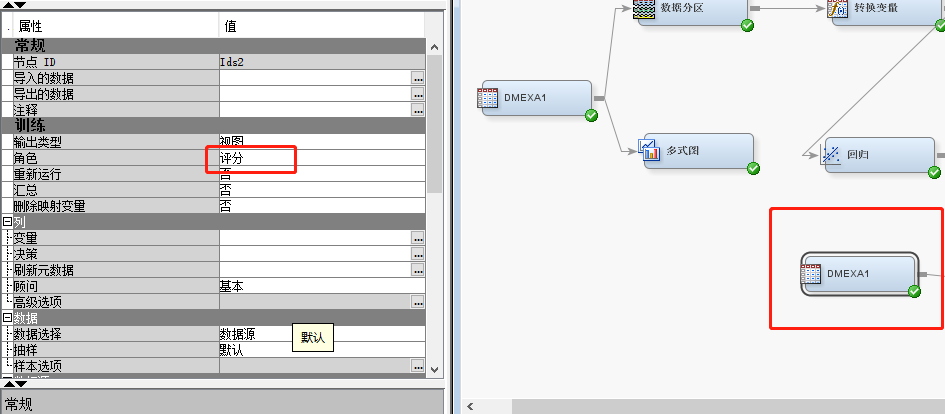拉入评分节点，运行，查看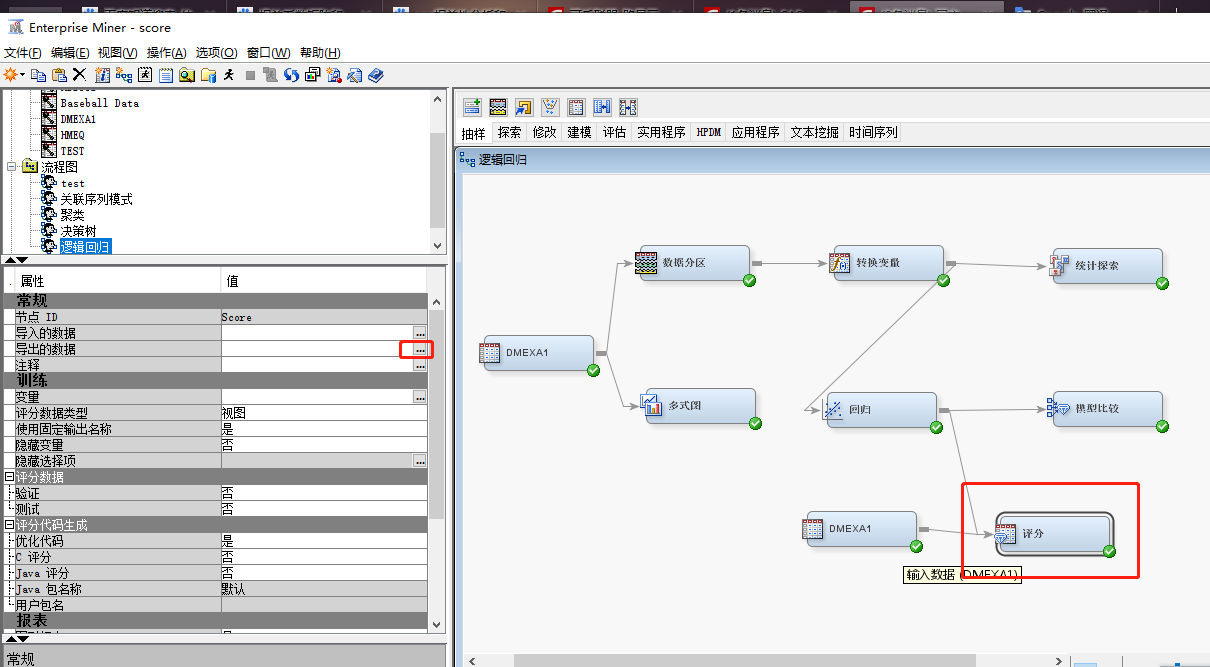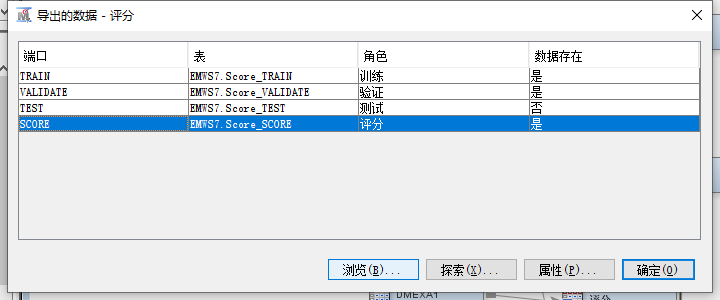查看预测结果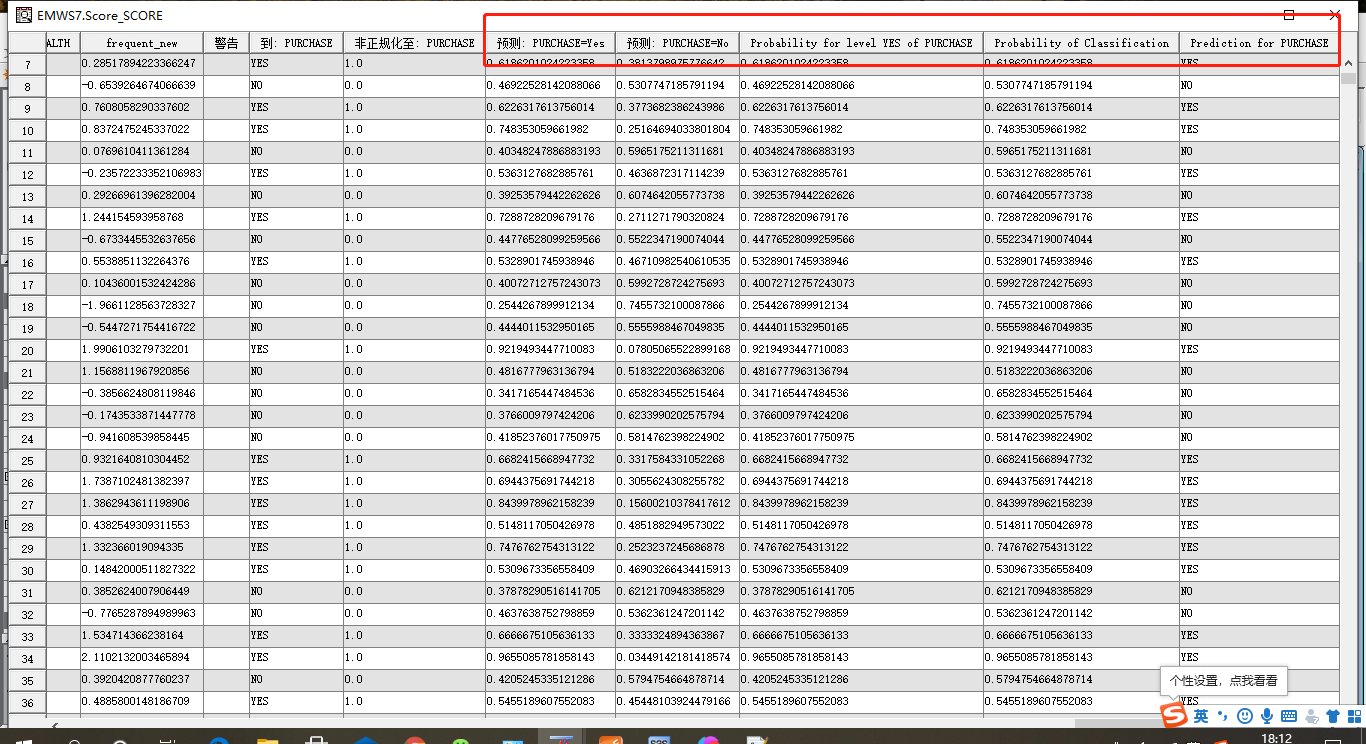文件保存到本地（sas代码节点）
拖入sas代码节点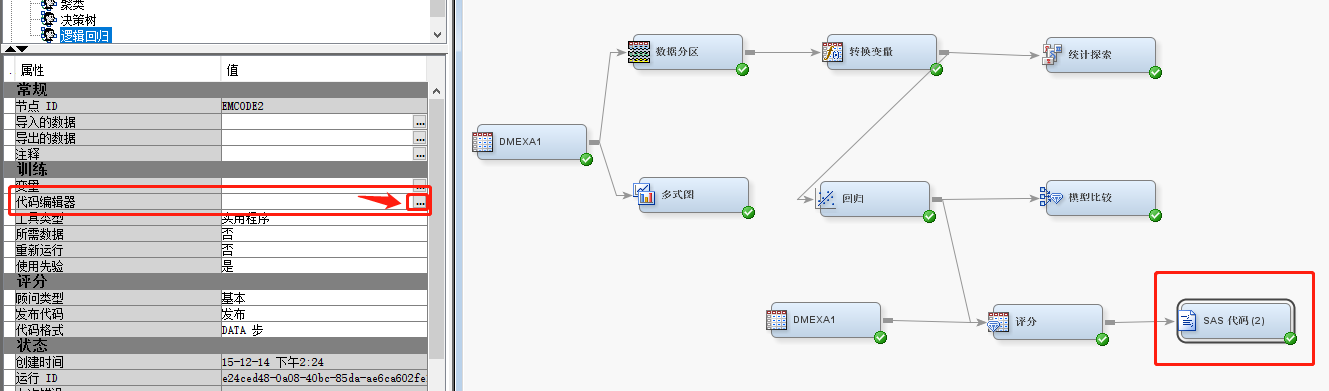编写sas代码
libname mylib "D:\score_data";
data mylib.score;
set EMWS7.Score_SCORE;
if P_PURCHASEYes LT 0.60 then delete;
run;
proc print data = mylib.score;
var  ACCTNUM P_PURCHASEYes;
run;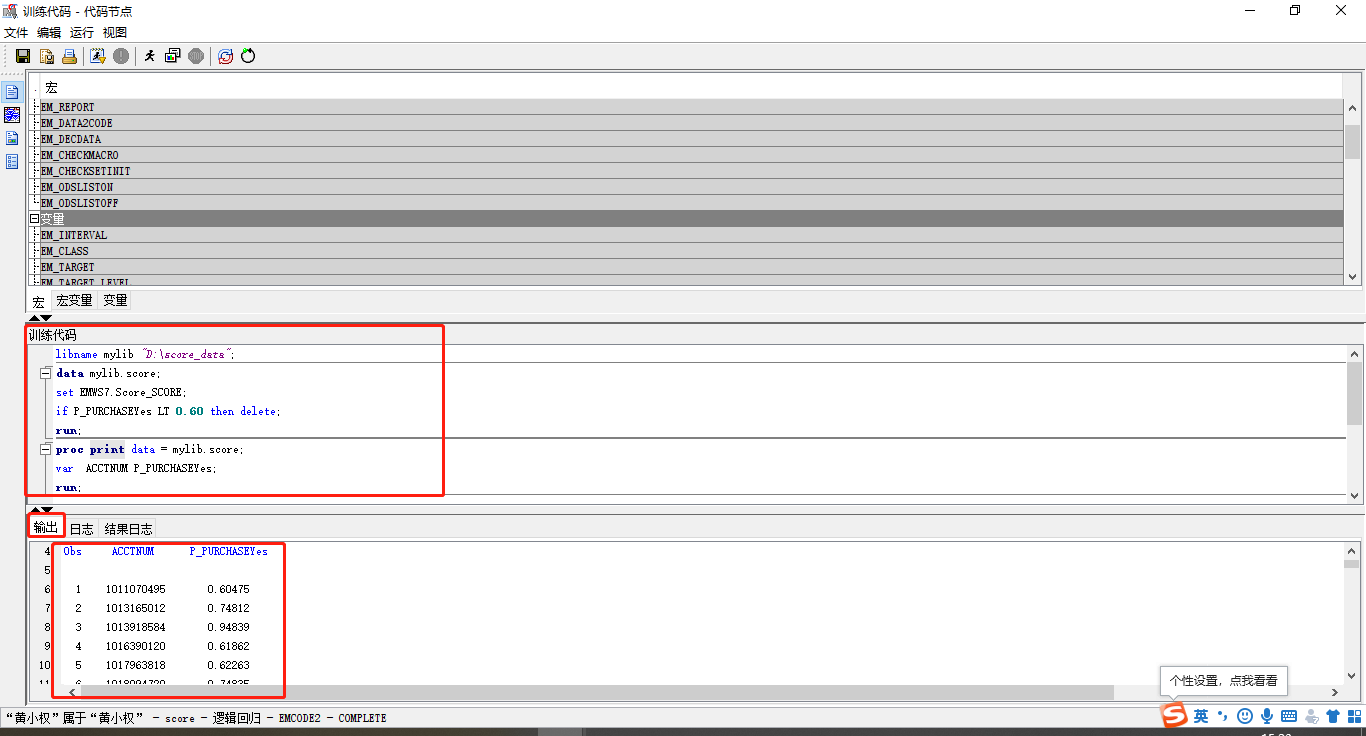查看本地文件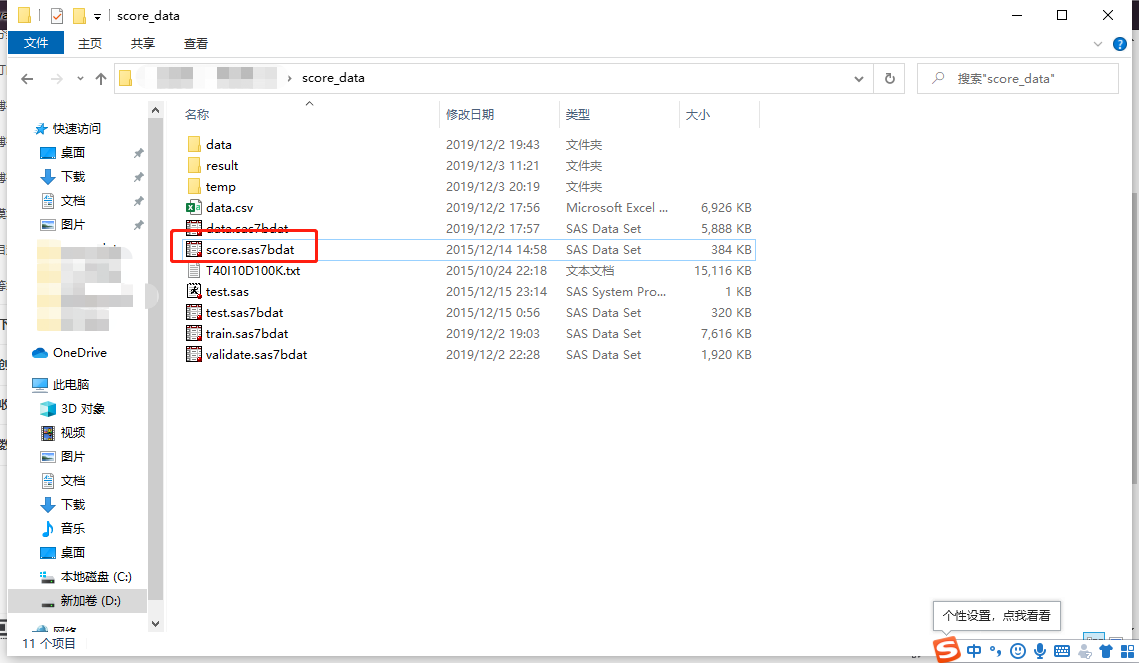展开全文• 学习笔记（二）逻辑回归预测贷款用户是否会逾期 算法实践能力的【整个思路】：构建模型——&gt;模型融合——&gt;模型评估——&gt;交叉验证——&gt;模型调参——&gt;特征工程。【遵循】一次只做...
学习笔记（二）逻辑回归预测贷款用户是否会逾期
算法实践能力的【整个思路】：构建模型——>模型融合——>模型评估——>交叉验证——>模型调参——>特征工程。【遵循】一次只做一件事，【先做再优化】的思路。每一个过程在完成任务的前提下，都可以拓展学习。【期望目标】掌握数据挖掘的流程，提升合作的能力。各位已经有经验，尤其是辅助的助教，还请多指导
给大家的是金融数据，我们要做的是预测贷款用户是否会逾期，表格中，status是标签：0表示未逾期，1表示逾期。要求：构建逻辑回归模型进行预测（在构建部分数据需要进行缺失值处理和数据类型转换，如果不能处理，可以直接暴力删除） 数据
数据处理
需要直接删除的数据 -Unnamed: 0 用户ID trade_no：不知道是什么，可以分析下 bank_card_no：卡号 id_name：名字 custid: ???需要离散化处理的数据（未处理好，待学习）针对日期数据的处理(转换成年月日)（未处理好，待学习）缺失值的填充归一化处理所有数据
代码展示
import numpy as np
import pandas as pd
from sklearn.metrics import classification_report
from sklearn.model_selection import train_test_split
from sklearn.preprocessing import StandardScaler
from sklearn.linear_model import LogisticRegression
from sklearn.linear_model import SGDClassifier
from sklearn.metrics import f1_score,mean_squared_error
"""
# 逻辑回归预测贷款用户是否会逾期
"""

"""
1.缺失值处理
"""
datanew=pd.DataFrame(datanew.fillna(0))
# 简单的缺失值处理
# datanew.replace(to_replace='0.', value=np.nan)
# 丢弃带有缺失值的数据
# datanew = datanew.dropna(axis=1, how='any')
data_columns = datanew.columns

"""
"""
dic = {}
for i, j in enumerate(n):
dic[j] = i
"""
1.3 数据集的切分
"""
X_train, X_test, y_train, y_test = train_test_split(datanew[data_columns[1:90]], datanew[data_columns],test_size=0.3, random_state=666)
# 临时将处理有难度的特征删去，留待日后处理
"""
1.4标准化数据，方差为1，均值为零
"""
standardScaler = StandardScaler()
X_train_fit = standardScaler.fit_transform(X_train)
X_test_fit = standardScaler.transform(X_test)

log_reg = LogisticRegression()
log_reg.fit(X_train_fit, y_train)
log_reg_predict = log_reg.predict(X_test_fit)
a=0
for i in log_reg_predict:
if i == 1:
a += 1
print("预测为1的个数：",a)
print("predict:",log_reg.score(X_test_fit, y_test))
print("f1_score:",f1_score(y_test, log_reg_predict, average='macro'))
print("mean_squared_error:",mean_squared_error(y_test, log_reg_predict))

结果：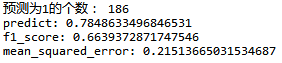问题
缺失值、日期、离散化数据的处理需要学习,数据处理的知识急需补充
展开全文算法
• 【原创】R语言逻辑回归预测分析付费用户数据分析报告论文（代码数据）.docxR语言
• 面对不同类型、偏好的消费者以及他们之前的消费数据作为基础，利用逻辑回归算法和随机森林回归算法构建模型，在已知数据的基础上进行拟合和调试，得出最优化的规律，并根据这一规律预测消费者的动机，此项研究在编程...python
• 逻辑回归算是机器学习中最基础的模型了，回归模型在做分类问题中有着较好的效果。下面介绍下利用sklearn做逻辑回归模型 做模型一般分为:提取数据---->了解数据(所谓的探索性数据)---->数据预处理(包括但不限于...
  逻辑回归算是机器学习中最基础的模型了，回归模型在做分类问题中有着较好的效果。下面介绍下利用sklearn做逻辑回归模型    做模型一般分为:提取数据---->了解数据(所谓的探索性数据)---->数据预处理(包括但不限于填充缺失值，特征提取，转换哑变量)---->选择模型---->验证模型---->模型优化
下面先简单介绍下逻辑回归的原理：
说到逻辑回归就不得不提一下线性回归，线性回归用wiki百科的定义来解释就是：在统计学中，线性回归是一种用来建立响应标量（因变量）和一个或多个解释变量（自变量）之间的模型关系的线性方法。线性回归分为一元线性回归和多元线性回归。均方误差是回归模型中常用的度量方法。一般用最小二乘法来最小化均方误差。
线性回归用的最多的是做预测，而逻辑回归最适合的有二分预测，比如是否垃圾邮件，广告是否点击等等；今天的模型用kaggle比赛中的泰坦尼克预测数据集来做逻辑回归模型，故此次我们做的是监督学习。
1.在数据集从kaggle中下载后我们先读取数据和数据预览：
通过DataFrame的函数info(),我们可以详细看到数据的分布等情况
import pandas as pd
print(train.info())  #show the information about train data,including counting values of null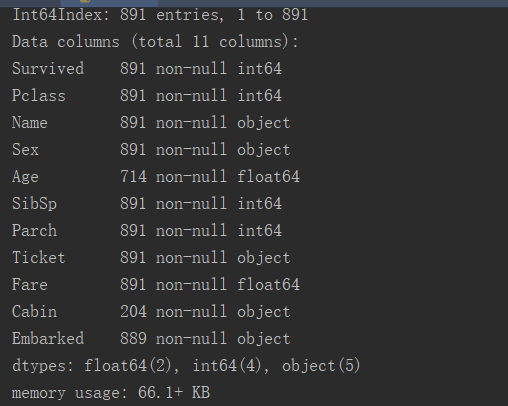2.了解数据：
查看数据中的缺失值
print(train.isnull().sum()发现数据中缺失数据Age有177个，Cabin 有687个，Embarked 有2个；由于Cabin 缺失数据占比太大了，我们看下这列数据缺失部分和有值部分对是否获救的情况是如何的，来判断该特征是否需要直接删除。
c=train.Cabin.value_counts() #get the value of Cabin
print(c)
train.drop(labels=Cabin,axis=1)
3.数据处理：
看了下结果发现都是一些客舱号，由于不具有很大的参考意义，在这里我直接把这个特征舍弃掉。
另一个列Embarked是登船的港口，有2个缺失值，我们用出现频率最多的值来填充
train.Embarked=train.Embarked.fillna('S')
Em=train.Embarked.value_counts()
print(Em)
接下来是Age有177个缺失值，
由于年龄和姓名相关，我们首先把未缺失的值按照姓名的简称来做下均值分类
train['cc']=train.Name.map(lambda x: str(re.compile(r',(.*)\.').findall(x)))#获取名字中的简称字样Mr,Miss,Mrs,Master,Dr等值
#替换上面的写法：train['cc']=train['Name'].apply(lambda x:x.split(',').split('.').strip())
c=train.loc[:,['cc','Age']].query('Age>0').groupby('cc').mean()  #按照名称辅助列看下各年龄的均值
最后我们看到结果如下：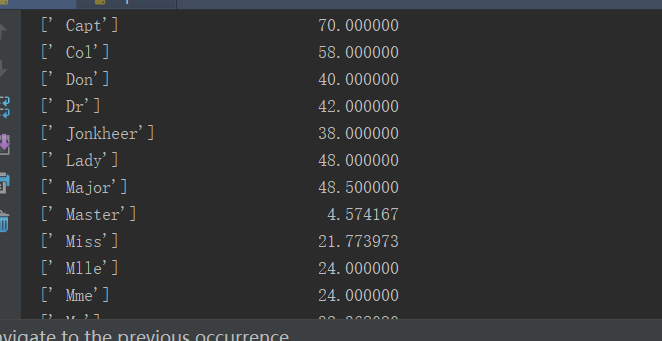在这里我们对缺失值的年龄填充根据姓名简称的均值来进行填充
train['Age']=train['Age'].fillna(0)#先对缺失值进行0填充
for i in range(1,891):
if train['Age'][i]==0 and train['cc'][i]=="[' Mr']":
train.loc[i, 'Age']=32
if train['Age'][i]==0 and train['cc'][i] =="[' Mrs']":
train.loc[i, 'Age']= 35
if train['Age'][i]==0 and train['cc'][i] == "[' Miss']":
train.loc[i, 'Age']=20
if train['Age'][i]==0 and train['cc'][i] == "[' Master']":
train.loc[i, 'Age']= 4
if train['Age'][i]==0 and train['cc'][i] == "[' Dr']":
train.loc[i,'Age']=42
另一种写法，如下：
value=['Mr','Miss','Mrs','Master','Dr']
for v in value:
train.loc[(train.Age==0)&(train.cc==v),'Age']=c.loc[v,'Age']
到这里我们就把缺失值处理完了，下面我们对类别值进行处理，我们先看下目前有哪些特征是类别
categore=train.dtypes[train.dtypes=='object'].index
结果为：我们看到，目前有年龄，船票和登船口是类别型的，这里我们对年龄和登船口做变量赋值
train=train.replace({'Sex':{'male':1,'female':2},
'Embarked':{'S':1,'C':2,'Q':3}}
)
后面我们把Name,Ticket等无意义的字段直接删掉
data=data.drop(labels=['cc','Name','Ticket'],axis=1)
到这里就数据处理完啦。由于kaggle数据都是直接把train,test给我们的，所以我一般会前期把train数据集和test数据集放一起做数据处理。这里前面我们可以在最初做数据拼接操作
data=pd.concat([train,test],keys=(['train','test']))
最后对所有的数据处理完后把数据分开
train_data=data.xs('train')#分开得到level 为train的测试数据
test_data=data.xs('test').drop(labels='Survived',axis=1)
x_train=train_data.drop(labels='Survived',axis=1)
y_train=train_data['Survived']
test_data=test_data.fillna(0)
4.选择模型
本次的特征较少，我们就不做特别的特征选取了， 对数据处理完后就直接进入模型阶段，这次我们在这里讲解Logistics回归模型。
首先对模型做均一化处理
from sklearn.preprocessing import StandardScaler
from sklearn.linear_model import LogisticRegression
S=StandardScaler()
S.fit(x_train)
x_train_stand=S.transform(x_train)
x_test_stand=S.transform(test_data)
Log=LogisticRegression(C=10)
Log.fit(x_train_stand,y_train)  #训练模型
prediction=Log.predict(x_test_stand)  #用训练的模型Log来预测测试数据
result=pd.DataFrame({'PassengerId':test_data.index,'Survived':prediction.astype(np.int32)})  #这里需要注意把prediction的数据转换成Int型不然系统判定不了，得分会为0
result.to_csv('D:\\pycm\\kaggle\\titanic\\result.csv',index=False) #设置不输出Index
最后将得到的结果提交，就完成啦，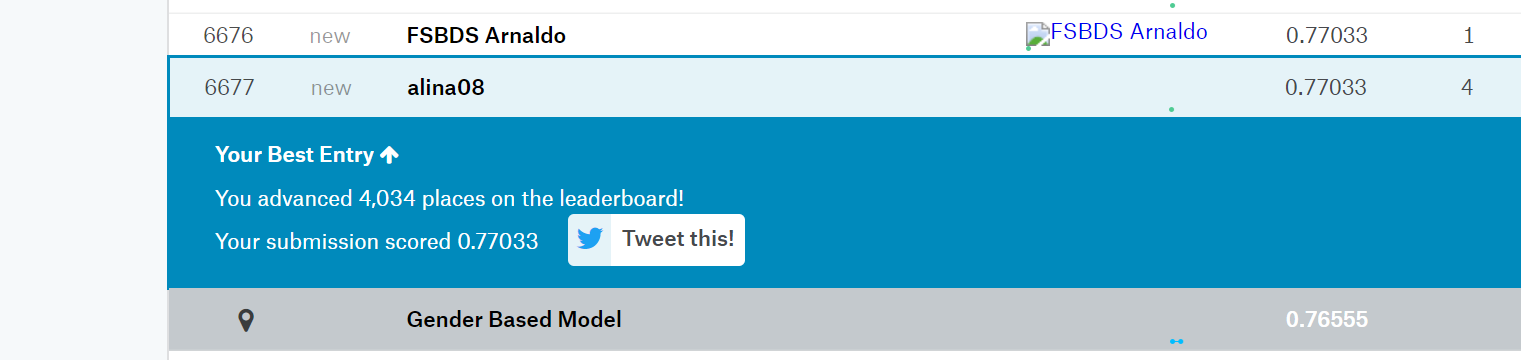后面还可以对模型进行优化，调参，我们放到下一期来进行讲解。这期讲解逻辑回归就先到这里，我们以一个是否生存的预测问题结尾。
最后附上完整代码：
import pandas as pd
import numpy as np
import seaborn as sns
import matplotlib
import matplotlib.pyplot as plt
import re
from sklearn.preprocessing import StandardScaler
from sklearn.linear_model import LogisticRegression
from sklearn.ensemble import RandomForestClassifier

data=pd.concat([train,test],keys=(['train','test']))
print(data.info())
data.Embarked=data.Embarked.fillna('S')
data=data.drop(labels='Cabin',axis=1)
#data['cc']=data.Name.map(lambda x: str(re.compile(r',(.*)\.').findall(x)))
data['cc']=data['Name'].apply(lambda x:x.split(',').split('.').strip())
c=data.loc[:,['cc','Age']].query('Age>0').groupby('cc').mean()
print(c.loc['Miss','Age'])
value=['Mr','Miss','Mrs','Master','Dr']
data['Age']=data['Age'].fillna(0)
for v in value:
data.loc[(data.Age==0)&(data.cc==v),'Age']=c.loc[v,'Age']
data=data.drop(labels=['cc','Name','Ticket'],axis=1)

data=data.replace({'Sex':{'male':1,'female':2},
'Embarked':{'S':1,'C':2,'Q':3}}
)

train_data=data.xs('train')
test_data=data.xs('test').drop(labels='Survived',axis=1)
x_train=train_data.drop(labels='Survived',axis=1)
y_train=train_data['Survived']
test_data=test_data.fillna(0)

S=StandardScaler()
S.fit(x_train)
x_train_stand=S.transform(x_train)
x_test_stand=S.transform(test_data)
Log=RandomForestClassifier(oob_score=True,random_state=10)
Log.fit(x_train_stand,y_train)
prediction=Log.predict(x_test_stand)
result=pd.DataFrame({'PassengerId':test_data.index,'Survived':prediction.astype(np.int32)})
result.to_csv('D:\\pycm\\kaggle\\titanic\\result.csv',index=False)


展开全文python sklearn
• 逻辑回归 房价预测的例子是很多机器学习课程的经典入门案例，房价受多种因素的影响，例如房屋面积、卧室数量等等，那么...房价是一个连续值，预测连续值的问题属于逻辑回归问题。借助python强大的机器学习类库，我...机器学习 波士顿房价 深度学习
• 用logistic回归预测糖尿病数据集_我在糖尿病数据集上使用了logistic回归和决策树分类器模型，在对两个模型进行训练和测试数据集比率相同后，我发现logistic回归给出的准确性更高，大约为80％，而决策树分类器给出了...
• 逻辑回归预测癌症分类 下面是用逻辑回归对癌症分类预测过程： #1获取数据 读取的时候加上names #2数据处理 处理缺失值 #3数据集划分 #4特征工程 标准化 #5逻辑回归预估器 #6模型评估 import pandas as pd import ...
• 最近学习预测，先从最简单的入手，本文写最近利用机器学习中的逻辑回归算法实现的两个实际案例： 1. 根据以往的申请表数据预测一个学生是否被大学录取 2. 信用卡欺诈预测 后边代码整理至我的github中，待续！！ ...机器学习
• 逻辑回归公式 hθ(x)=g(θTx)=11+e−θTxh_\theta(x) = g(\theta^Tx)=\frac{1}{1+e^{-\theta^Tx}}hθ​(x)=g(θTx)=1+e−θTx1​ g(z)=11+e−zg(z) = \frac{1}{1+e^{-z}}g(z)=1+e−z1​ sigmoid函数g(z) 过点：(0, ...
• 上一次发的那个回归是线性回归，这次给大家发一下逻辑回归 原数据 from pyspark.mllib.linalg import Vectors,Vector from pyspark import SparkContext from pyspark.ml.regression import LinearRegression from...
• rlr = RLR() #建立随机逻辑回归模型，筛选变量 rlr.fit(x, y) #训练 rlr.get_support() #获取特征结果 print(u'通过随机逻辑回归筛选特征结束') print('有效特征为' +','.join(list(data.columns[rlr.get_support()]...
• from sklearn.linear_model import LogisticRegression # 实例化模型 lr = LogisticRegression(C=c_value, penalty='l1', solver='liblinear') # 训练模型 lr.fit(x_train, y_train....# 模型预测 y_predict = lr.pr...python 机器学习
• 如果是二元逻辑回归，ovr和multinomial并没有任何区别，区别主要在多元逻辑回归上。 ovr不论是几元回归，都当成二元回归来处理。mvm从从多个类中每次选两个类进行二元回归。如果总共有T类，需要T(T-1)/2次分类。 ...sklearn logistic gridsearch
• 1，训练样本 mahout trainlogistic --input dataset/donut.csv \ --output ./model \ --target color --categories 2 \ --predictors x y --types numeric \ -- features 20 --passes 100 --rate 50 ...
• 废话不多说，直接上代码 train = pd.read_csv('train_xy.csv') traint, testst = train_test_split(train, test_size=0.4, random_state=1)#分割数据集 params = { 'booster': 'gbtree', ...
• 现在我这里有一份数据集，数据量很少，只有不到200行，里面有0和1的标签，很适合做二分类，数据集如下： LOW,AGE,LWT,RACE,SMOKE,PTL,HT,UI,BWT 1,28,113,1,1,1,0,1,709 1,29,130,0,0,0,0,1,1021 ...
• LogisticRegression(C=1.0, class_weight=None, dual=False, fit_intercept=True, intercept_scaling=1, max_iter=100, multi_class='ovr', n_jobs=1, penalty='l1', random_state=None, solver='liblinear', tol=...shell...